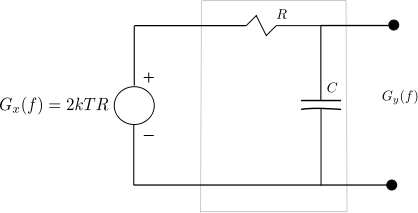- DSP log - http://www.dsplog.com -

Thermal noise of RC low pass filter

Posted By Krishna Sankar On May 6, 2012 @ 8:48 am In Analog | 1 Comment

This post discuss about the thermal noise in RC low pass filter. Using the noise equivalent model using resistor with a voltage source, which gets passed through a no noise RC low pass filter. The noise power at the output is computed by integrating the output voltage spectral density over all frequencies.

Consider a simple RC low pass filter with resistor  and capacitance  as shown in the figure below.Figure : Noise equivalent model of RC low pass filter (Reference : Figure 9.3-6 in Communication Systems – An introduction to Signals and noise in Electrical Communication by A. Bruce Carlson, Paul Crilly, Janet Rutledge )

As discussed in the post on thermal noise and AWGN , the resistor  is generating a noise having power  having power spectral density

.

The noise voltage gets applied to a noise less RC low pass filter having transfer function,

, where

is the -3dB bandwidth of the filter.

The power spectral density of the output signal is,

.

Integrating the output power spectral density over all frequencies from   will give the noise voltage at the output, i.e.

.

Computing  by plugging in the expression for ,

.

(Note: The neat trick behind integration described below)

Plugging in  and , the noise power at the output is,

.

Summarizing, the mean square voltage at the output of the RC low pass filter is,

.

Plugging in capacitance values from 1pF to 1nF, the root mean square voltage is shown below.

 k (Boltzmann constant) 1.38E-023 Joules/Kelvin T (temperature) 300 Kelvin Capacitance, farad RMS voltage, V 1.00E-012 6.434E-005 1.00E-011 2.035E-005 1.00E-010 6.434E-006 1.00E-009 2.035E-006

Observations:

a) As expected from the equation, when the capacitance is increased, the noise voltage reduces (as the filter bandwidth is getting narrower).

b) The noise power at the output is independent of resistor . Increasing the resistance will increase the noise power , but decreases the filter bandwidth . Similarly decreasing the resistor will reduce the noise power, but increases the filter bandwidth. These two effects cancel each other leaving the noise power dependent only on the temperature  and capacitance .

References:

Note :

Liked the neat trick using  substitution for solving the integration over all frequencies in a first order RC low pass filter. Did some googling to find that out…and the steps are as follows.

Let , then   and the integration limits are

.

Also, note that (thanks to the List of Trigonometric Identities from wikipedia , Differentiation rules from wikipedia )

,

Plugging all the above,

. Neat!

URL to article: http://www.dsplog.com/2012/05/06/thermal-noise-rc-low-pass-filter/

URLs in this post:

 Communication Systems – An introduction to Signals and noise in Electrical Communication by A. Bruce Carlson, Paul Crilly, Janet Rutledge: http://www.amazon.com/gp/redirect.html?ie=UTF8&location=http%3A%2F%2Fwww.amazon.com%2FCommunication-Systems-Bruce-Carlson%2Fdp%2F0070111278&tag=dl04-20&linkCode=ur2&camp=1789&creative=9325

 thermal noise and AWGN: http://www.dsplog.com/2012/03/25/thermal-noise-awgn/

 List of Trigonometric Identities from wikipedia: http://en.wikipedia.org/wiki/List_of_trigonometric_identities

 Differentiation rules from wikipedia: http://en.wikipedia.org/wiki/Differentiation_rules

 Johnson-Nyquist Noise from wikipedia: http://en.wikipedia.org/wiki/Johnson%E2%80%93Nyquist_noise